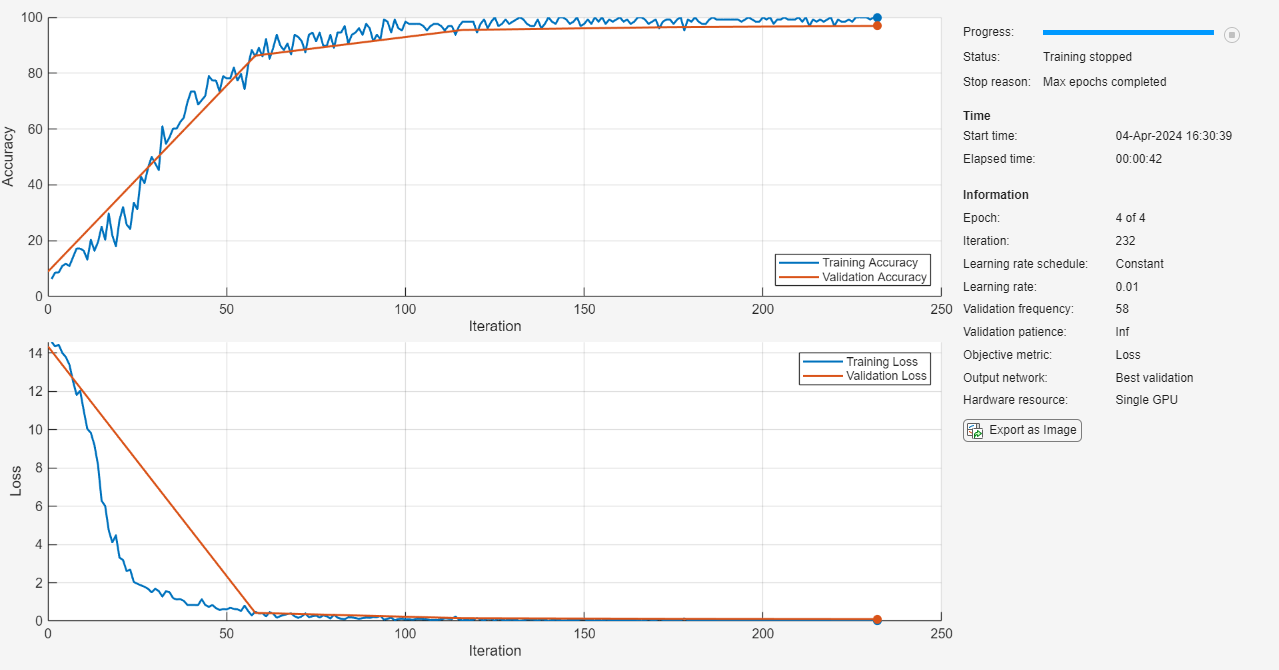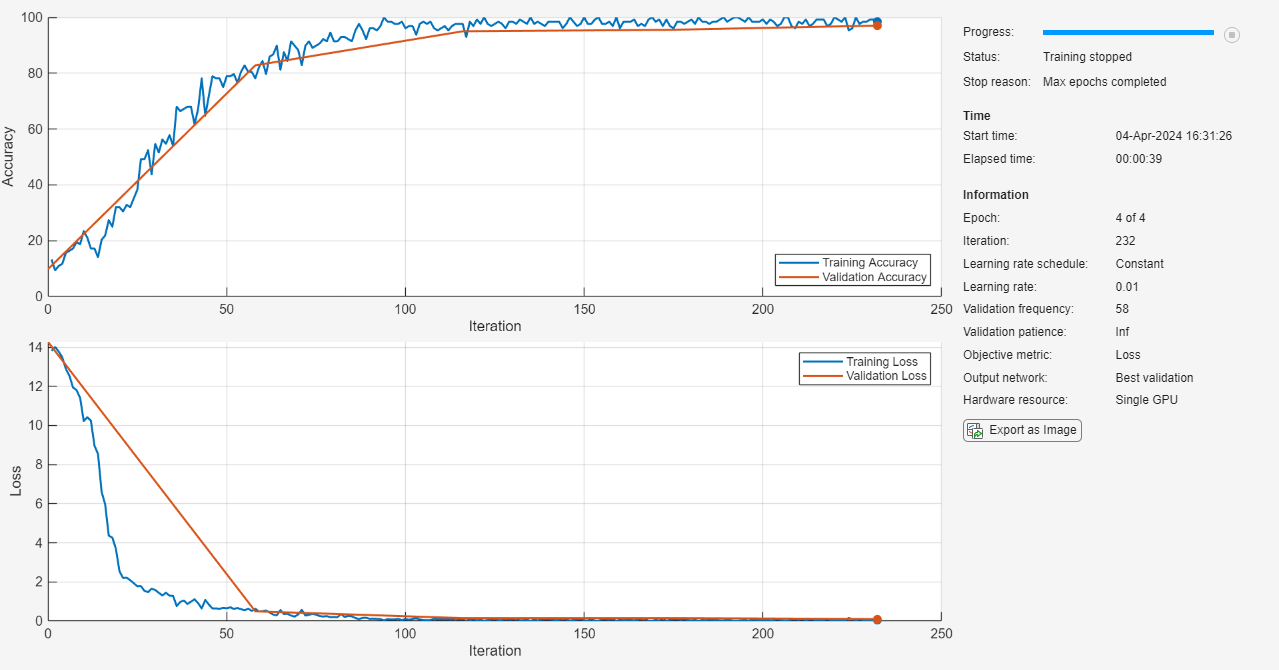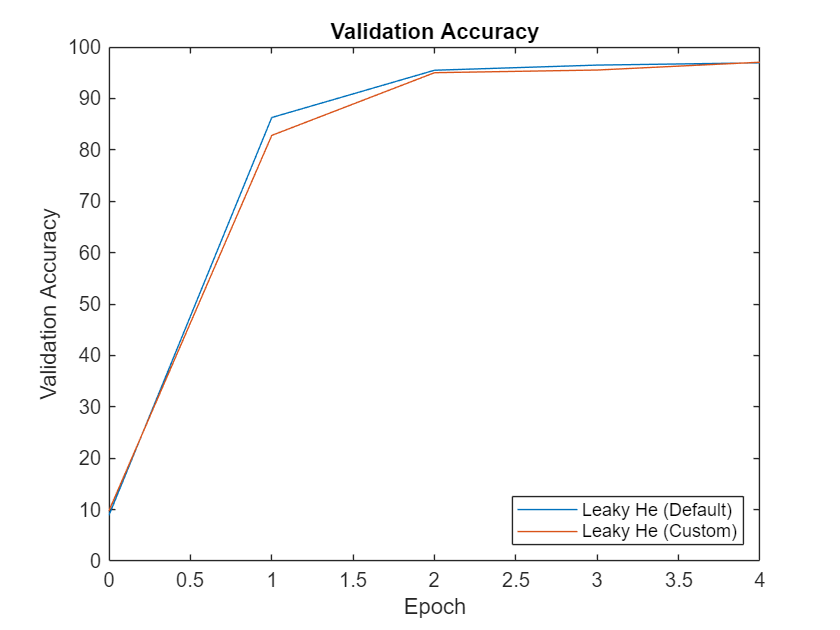Main Content

# Specify Custom Weight Initialization Function

This example shows how to create a custom He weight initialization function for convolution layers followed by leaky ReLU layers.

The He initializer for convolution layers followed by leaky ReLU layers samples from a normal distribution with zero mean and variance ${\sigma }^{2}=\frac{2}{\left(1+{\mathit{a}}^{2}\right)\mathit{n}}$, where a is the scale of the leaky ReLU layer that follows the convolution layer and `n = FilterSize(1) * FilterSize(2) * NumChannels`.

For learnable layers, when setting the options `'WeightsInititializer'`, `'InputWeightsInitializer'`, or `'RecurrentWeightsInitializer'` to `'he'`, the software uses a=0. To set a to different value, create a custom function to use as a weights initializer.

### Load Data

Load the digit sample data as an image datastore. The `imageDatastore` function automatically labels the images based on folder names.

```digitDatasetPath = fullfile(matlabroot,'toolbox','nnet','nndemos', ... 'nndatasets','DigitDataset'); imds = imageDatastore(digitDatasetPath, ... 'IncludeSubfolders',true, ... 'LabelSource','foldernames');```

Divide the data into training and validation data sets, so that each category in the training set contains 750 images, and the validation set contains the remaining images from each label. `splitEachLabel` splits the datastore into two new datastores for training and validation.

```numTrainFiles = 750; [imdsTrain,imdsValidation] = splitEachLabel(imds,numTrainFiles,'randomize');```

### Define Network Architecture

Define the convolutional neural network architecture:

• Image input layer size of `[28 28 1]`, the size of the input images

• Three 2-D convolution layers with filter size 3 and with 8, 16, and 32 filters respectively

• A leaky ReLU layer following each convolutional layer

• Fully connected layer of size 10, the number of classes

• Softmax layer

• Classification layer

For each of the convolutional layers, set the weights initializer to the `leakyHe` function. The `leakyHe` function, listed at the end of the example, takes the input `sz` (the size of the layer weights) and returns an array of weights given by the He Initializer for convolution layers followed by a leaky ReLU layer.

```inputSize = [28 28 1]; numClasses = 10; layers = [ imageInputLayer(inputSize) convolution2dLayer(3,8,'WeightsInitializer',@leakyHe) leakyReluLayer convolution2dLayer(3,16,'WeightsInitializer',@leakyHe) leakyReluLayer convolution2dLayer(3,32,'WeightsInitializer',@leakyHe) leakyReluLayer fullyConnectedLayer(numClasses) softmaxLayer classificationLayer];```

### Train Network

Specify the training options and train the network. Train for four epochs. To prevent the gradients from exploding, set the gradient threshold to 2. Validate the network once per epoch. View the training progress plot.

By default, `trainNetwork` uses a GPU if one is available, otherwise, it uses a CPU. Training on a GPU requires Parallel Computing Toolbox™ and a supported GPU device. For information on supported devices, see GPU Support by Release (Parallel Computing Toolbox). You can also specify the execution environment by using the `'ExecutionEnvironment'` name-value pair argument of `trainingOptions`.

```maxEpochs = 4; miniBatchSize = 128; numObservations = numel(imdsTrain.Files); numIterationsPerEpoch = floor(numObservations / miniBatchSize); options = trainingOptions('sgdm', ... 'MaxEpochs',maxEpochs, ... 'MiniBatchSize',miniBatchSize, ... 'GradientThreshold',2, ... 'ValidationData',imdsValidation, ... 'ValidationFrequency',numIterationsPerEpoch, ... 'Verbose',false, ... 'Plots','training-progress'); [netDefault,infoDefault] = trainNetwork(imdsTrain,layers,options);```### Test Network

Classify the validation data and calculate the classification accuracy.

```YPred = classify(netDefault,imdsValidation); YValidation = imdsValidation.Labels; accuracy = mean(YPred == YValidation)```
```accuracy = 0.9684 ```

### Specify Additional Options

The `leakyHe` function accepts the optional input argument `scale`. To input extra variables into the custom weight initialization function, specify the function as an anonymous function that accepts a single input `sz`. To do this, replace instances of `@leakyHe` with `@(sz) leakyHe(sz,scale)`. Here, the anonymous function accepts the single input argument `sz` only and calls the `leakyHe` function with the specified `scale` input argument.

Create and train the same network as before with the following changes:

• For the leaky ReLU layers, specify a scale multiplier of 0.01.

• Initialize the weights of the convolutional layers with the `leakyHe` function and also specify the scale multiplier.

```scale = 0.01; layers = [ imageInputLayer(inputSize) convolution2dLayer(3,8,'WeightsInitializer',@(sz) leakyHe(sz,scale)) leakyReluLayer(scale) convolution2dLayer(3,16,'WeightsInitializer',@(sz) leakyHe(sz,scale)) leakyReluLayer(scale) convolution2dLayer(3,32,'WeightsInitializer',@(sz) leakyHe(sz,scale)) leakyReluLayer(scale) fullyConnectedLayer(numClasses) softmaxLayer classificationLayer]; [netCustom,infoCustom] = trainNetwork(imdsTrain,layers,options);```Classify the validation data and calculate the classification accuracy.

```YPred = classify(netCustom,imdsValidation); YValidation = imdsValidation.Labels; accuracy = mean(YPred == YValidation)```
```accuracy = 0.9456 ```

### Compare Results

Extract the validation accuracy from the information structs output from the `trainNetwork` function.

```validationAccuracy = [ infoDefault.ValidationAccuracy; infoCustom.ValidationAccuracy];```

The vectors of validation accuracy contain `NaN` for iterations that the validation accuracy was not computed. Remove the `NaN` values.

```idx = all(isnan(validationAccuracy)); validationAccuracy(:,idx) = [];```

For each of the networks, plot the epoch numbers against the validation accuracy.

```figure epochs = 0:maxEpochs; plot(epochs,validationAccuracy) title("Validation Accuracy") xlabel("Epoch") ylabel("Validation Accuracy") legend(["Leaky He (Default)" "Leaky He (Custom)"],'Location','southeast')```### Custom Weight Initialization Function

The `leakyHe` function takes the input `sz` (the size of the layer weights) and returns an array of weights given by the He Initializer for convolution layers followed by a leaky ReLU layer. The function also accepts the optional input argument `scale` which specifies the scale multiplier for the leaky ReLU layer.

```function weights = leakyHe(sz,scale) % If not specified, then use default scale = 0.1 if nargin < 2 scale = 0.1; end filterSize = [sz(1) sz(2)]; numChannels = sz(3); numIn = filterSize(1) * filterSize(2) * numChannels; varWeights = 2 / ((1 + scale^2) * numIn); weights = randn(sz) * sqrt(varWeights); end```

### Bibliography

1. He, Kaiming, Xiangyu Zhang, Shaoqing Ren, and Jian Sun. "Delving deep into rectifiers: Surpassing human-level performance on imagenet classification." In Proceedings of the IEEE international conference on computer vision, pp. 1026-1034. 2015.

Download ebook